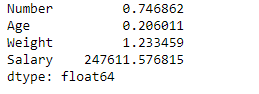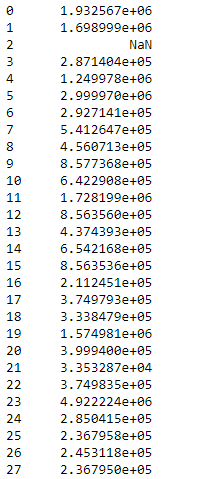Open in App
Not now

# Python | Pandas dataframe.sem()

• Last Updated : 23 Nov, 2018

Python is a great language for doing data analysis, primarily because of the fantastic ecosystem of data-centric python packages. Pandas is one of those packages and makes importing and analyzing data much easier.

Pandas` dataframe.sem()` function return unbiased standard error of the mean over requested axis. The standard error (SE) of a statistic (usually an estimate of a parameter) is the standard deviation of its sampling distribution or an estimate of that standard deviation. If the parameter or the statistic is the mean, it is called the standard error of the mean (SEM).

Syntax : DataFrame.sem(axis=None, skipna=None, level=None, ddof=1, numeric_only=None, **kwargs)

Parameters :
axis : {index (0), columns (1)}
skipna : Exclude NA/null values. If an entire row/column is NA, the result will be NA
level : If the axis is a MultiIndex (hierarchical), count along a particular level, collapsing into a Series
ddof : Delta Degrees of Freedom. The divisor used in calculations is N – ddof, where N represents the number of elements.
numeric_only : Include only float, int, boolean columns. If None, will attempt to use everything, then use only numeric data. Not implemented for Series

Return : sem : Series or DataFrame (if level specified)

Example #1: Use `sem()` function to find the standard error of the mean of the given dataframe over the index axis.

 `# importing pandas as pd ` `import` `pandas as pd ` ` `  `# Creating the dataframe  ` `df ``=` `pd.read_csv(``"nba.csv"``) ` ` `  `# Print the dataframe ` `df `Let’s use the `dataframe.sem()` function to find the standard error of the mean over the index axis.

 `# find standard error of the mean of all the columns ` `df.sem(axis ``=` `0``) `

Output :Notice, all the non-numeric columns and values are automatically not included in the calculation of the dataframe. We did not have to specifically input the numeric columns for the calculation of the standard error of the mean.

Example #2: Use `sem()` function to find the standard error of the mean over the column axis. Also do not skip the `NaN` values in the calculation of the dataframe.

 `# importing pandas as pd ` `import` `pandas as pd ` ` `  `# Creating the dataframe  ` `df ``=` `pd.read_csv(``"nba.csv"``) ` ` `  `# Calculate the standard error of  ` `# the mean of all the rows in dataframe ` `df.sem(axis ``=` `1``, skipna ``=` `False``) `

Output :When we include the `NaN` values then it will cause that particular row or column to be `NaN`

My Personal Notes arrow_drop_up
Related Articles